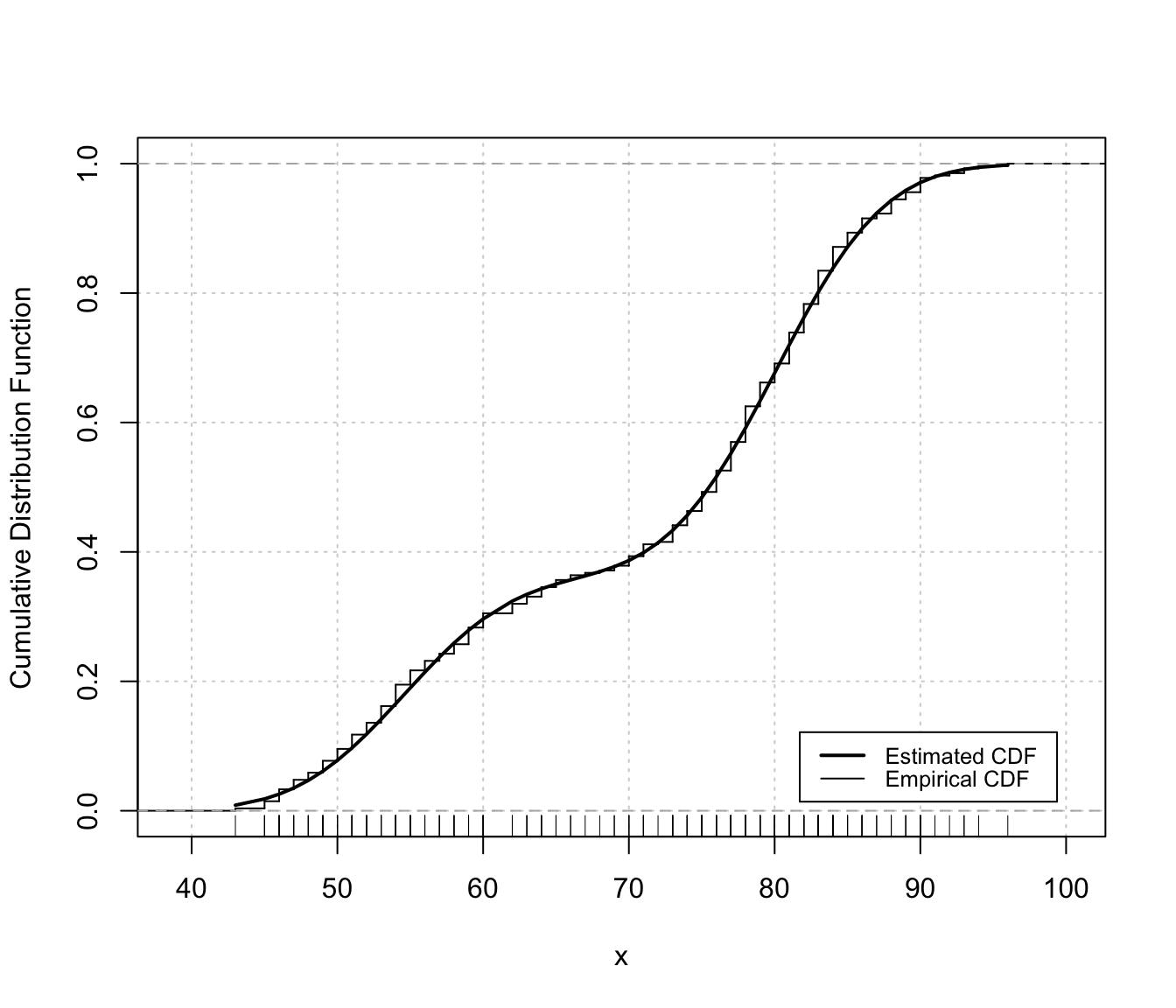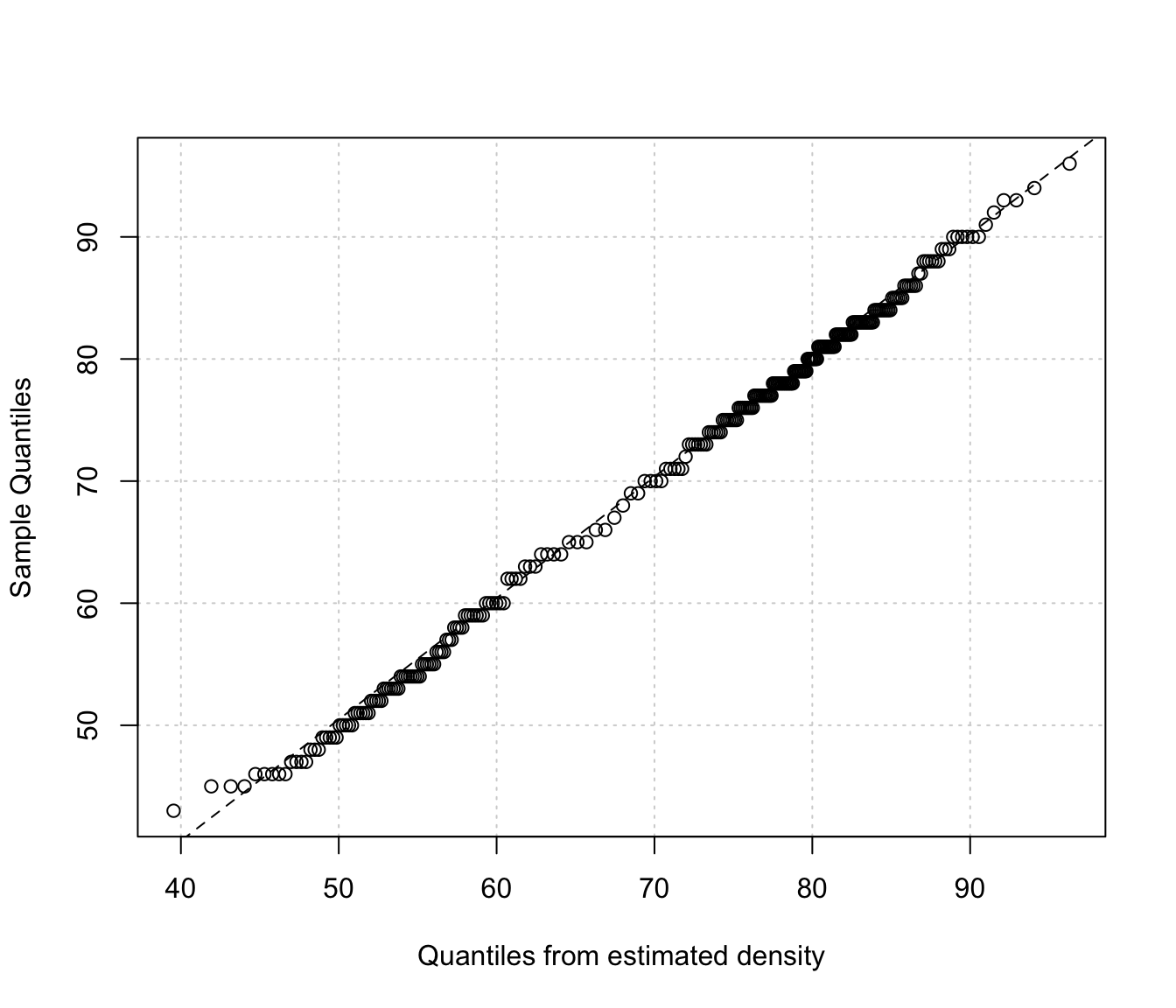Diagnostic plots for density estimation. Only available for the one-dimensional case.

densityMclust.diagnostic(object, type = c("cdf", "qq"),
col = c("black", "black"),
lwd = c(2,1), lty = c(1,1),
legend = TRUE, grid = TRUE,
...)

Arguments

object

An object of class 'mclustDensity' obtained from a call to densityMclust function.

type

The type of graph requested:

"cdf" =

a plot of the estimated CDF versus the empirical distribution function.

"qq" =

a Q-Q plot of sample quantiles versus the quantiles obtained from the inverse of the estimated cdf.

col

A pair of values for the color to be used for plotting, respectively, the estimated CDF and the empirical cdf.

lwd

A pair of values for the line width to be used for plotting, respectively, the estimated CDF and the empirical cdf.

lty

A pair of values for the line type to be used for plotting, respectively, the estimated CDF and the empirical cdf.

legend

A logical indicating if a legend must be added to the plot of fitted CDF vs the empirical CDF.

grid

A logical indicating if a grid should be added to the plot.

...

Details

The two diagnostic plots for density estimation in the one-dimensional case are discussed in Loader (1999, pp- 87-90).

Luca Scrucca

Examples

# \donttest{
x <- faithful\$waiting
dens <- densityMclust(x)plot(dens, x, what = "diagnostic")# or
densityMclust.diagnostic(dens, type = "cdf")densityMclust.diagnostic(dens, type = "qq")# }